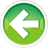# 1.8 — Introduction to literals and operators

Literals

Consider the following two statement:

What are “Hello world!” and 5? They are literals. A literal (also known as a literal constant) is a fixed value that has been inserted directly into the source code.

Literals and variables both have a value (and a type). However, the value of a literal is fixed and can’t be changed (hence it being called a constant), whereas the value of a variable can be changed through initialization and assignment.

Operators

In mathematics, an operation is a mathematical calculation involving zero or more input values (called operands) that produces a new value (called an output value). The specific operation to be performed is denoted by a construct (typically a symbol or pair of symbols) called an operator.

For example, as children we all learn that 2 + 3 equals 5. In this case, the literals 2 and 3 are the operands, and the symbol + is the operator that tells us to apply mathematical addition on the operands to produce the new value 5.

Author's note

For reasons that will become clear when we discuss operators in more detail, for operators that are symbols, it is common nomenclature to append the operator’s symbol to the word operator.

For example, the plus operator would be called operator+, and the minus operator would be called operator-.

You are likely already quite familiar with standard arithmetic operators from common usage in mathematics, including addition (+), subtraction (-), multiplication (*), and division (/). In C++, assignment (=) is an operator as well. Some operators use more than one symbol, such as the equality operator (==), which allows us to compare two values to see if they are equal. There are also a number of operators that are words (e.g. new, delete, and throw).

The number of operands that an operator takes as input is called the operator’s arity (almost nobody knows what this word means, so don’t drop it in a conversation and expect anybody to have any idea what you’re talking about). Operators in C++ come in three different arities:

Unary operators act on one operand. An example of a unary operator is the - operator. For example, given `-5`, operator- takes literal operand 5 and flip its sign to produce new output value -5.

Binary operators act on two operands (known as left and right). An example of a binary operator is the + operator. For example, given `3 + 4`, operator+ takes the left operand (3) and the right operand (4) and applies mathematical addition to produce new output value 7.

Ternary operators act on three operands. There is only one of these in C++, which we’ll cover later.

Note that some operators have more than one meaning depending on how they are used. For example, operator- has two contexts. It can be used in unary form to invert a number’s sign (e.g. to convert 5 to -5, or vice versa), or it can be used in binary form to do subtraction (e.g. 4 - 3).

Chaining operators

Operators can be chained together such that the output of one operator can be used as the input for another operator. For example, given the following: 2 * 3 + 4, the multiplication operator goes first, and converts left operand 2 and right operand 3 into new value 6 (which becomes the left operand for the plus operator). Next, the plus operator executes, and converts left operand 6 and right operand 4 into new value 10.

We’ll talk more about the order in which operators execute when we do a deep dive into the topic of operators. For now, it’s enough to know that the arithmetic operators execute in the same order as they do in standard mathematics: Parenthesis first, then Exponents, then Multiplication & Division, then Addition & Subtraction. This ordering is sometimes abbreviated PEDMAS, or the mnemonic “Please Excuse My Dear Aunt Sally”.

Quiz time

Question #1

For each of the following, indicate what output they produce:

a)

Show Solution

b)

Show Solution

c)

Show Solution1.9 -- Introduction to expressionsIndex1.7 -- Keywords and naming identifiers